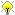[Documentation] [TitleIndex] [WordIndex]Please ask about problems and questions regarding this tutorial on answers.ros.org. Don't forget to include in your question the link to this page, the versions of your OS & ROS, and also add appropriate tags.

# Understanding the motor model

Description: Understanding motor model checks, and motor trace.

Tutorial Level: INTERMEDIATE

## Introduction

All of the PR2 motor control board (MCB) drivers use an electrical model brushed motors to detect problems. Using this electrical model, the software can detected the following problems:

• major encoder problems
• unplugged or missing encoder
• motor short circuit
• motor open circuit
• misbehaving motor control board
• wrong motor

The model so it can NOT detect issues like:

• mechanical damage
• encoder drift
• encoder that that is only slightly damaged

## Electrical Model

The following model of an electrical motor is used:

• `voltage = current*resistance + Kv*velocity`

The equation can be re-arranged to sum to zero:

• `current*resistance + Kv*velocity - voltage = 0`

For a ideal motor and ideal sensors the software could just make sure above equation equals zero.

• ```error = current*resistance + Kv*velocity - voltage
if (error != 0)
{
halt_motors()
}```

Since all motors parameters and measurements have variation or noise, the following check is used to detect a problem if motor:

• ```error = abs(current*resistance + Kv*velocity - voltage)
filtered_error = IIR_filter(error)
if ( filtered_error > limit )
{
halt_motors()
}```

## Determining Problem

The motor model equation can be used to detect that is something is wrong, but it can't determine what caused the problem. There are some simple heuristics that the motor model uses to guess the cause. But these heuristics don't always work.

### Detection heuristic example

If the encoder because unplugged, the measured velocity would be zero. So one possible hueristic would be to blame encoder if velocity was zero when motor model error was detected :

• ```error = abs(current*resistance + Kv*velocity - voltage)
filtered_error = IIR_filter(error)
if ( filtered_error > limit )
{
halt_motors()
if (velocity == 0)
{
print("Encoder might be damaged");
}
else
{
print("Problem with motor or encoder");
}
}```

While the above code might work sometimes, their might be other reason why the encoder might not be moving:

• The motor is mechanically being held in place.
• The motor is unplugged (and no outside forces are being applied)
• etc...

Many times it is easier to detect motor problems using outside information. If I manually move a joint I know the encoder velocity should not be zero. Using this outside information I can better determine the problem. Unfortunately, we don't always have physical access to a robot.

## Motor Trace

Some motor problems only for short period of time. For instance, a loose connector could disconnect motor for only short period of time because due a certain movement that would be difficult to reproduce. The motor model would report an error, but would not be able to determine what caused error later. For this reason (and others) a motor "trace" is published when motor halts due to error. The motor trace contains all data of motor that occurred a second before an error was detected.

On the PR2 robot the motor trace is recorded along with diagnostics to a bag file. By looking at the motor trace data it is sometimes possible to determine what the problem with a motor might have been.

The mtrace_plot tool can be used to plot motor trace data.

2020-02-22 12:41